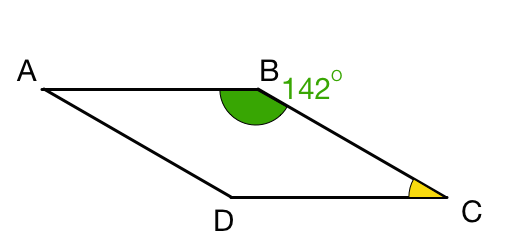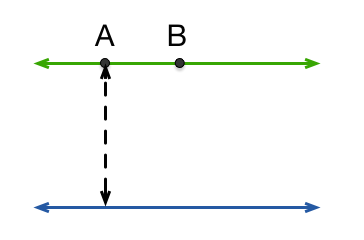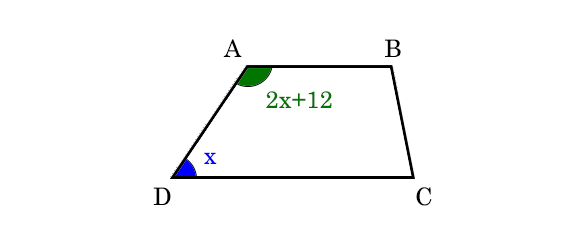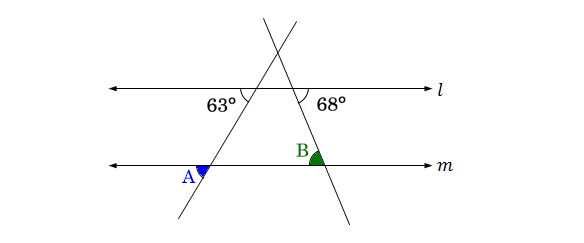Everyday Math

# Parallel LinesIn parallelogram $ABCD$, we are given that the green angle $\angle ABC = 142 ^ \circ$. What is the yellow angle $\angle BCD$?The green and blue lines are parallel, and A and B are points on the green line. If the shortest distance from A to the blue line is 5, what can we say about the shortest distance from B to the blue line?If $l \parallel m$, and $\angle a = 21^\circ$, what is the measure of $\angle b$ in degrees?Trapezoid $ABCD$ has $\angle D = \color{#3D99F6}{x}$ and $\angle A = \color{#20A900}{2x + 12^\circ}$. What is the measure of $\angle D$?In the above diagram, if $l \parallel m,$ what is the measure of $\color{#3D99F6}{\angle A}+\color{#20A900}{\angle B} ?$

×